Thank you for visiting nature.com. You are using a browser version with limited support for CSS. To obtain the best experience, we recommend you use a more up to date browser (or turn off compatibility mode in Internet Explorer). In the meantime, to ensure continued support, we are displaying the site without styles and JavaScript.

# Mobility transition from ballistic to diffusive transport in non-Hermitian lattices

## Abstract

Within all physical disciplines, it is accepted that wave transport is predetermined by the existence of disorder. In this vein, it is known that ballistic transport is possible only when a structure is ordered, and that disorder is crucial for diffusion or (Anderson-)localization to occur. As this commonly accepted picture is based on the very foundations of quantum mechanics where Hermiticity of the Hamiltonian is naturally assumed, the question arises whether these concepts of transport hold true within the more general context of non-Hermitian systems. Here we demonstrate theoretically and experimentally that in ordered time-independent -symmetric systems, which are symmetric under space-time reflection, wave transport can undergo a sudden change from ballistic to diffusive after a specific point in time. This transition as well as the diffusive transport in general is impossible in Hermitian systems in the absence of disorder. In contrast, we find that this transition depends only on the degree of dissipation.

## Introduction

The deceleration of wave transport in a lattice due to disorder was introduced in physics about 100 years ago with the famous Drude model. Paul Drude explained the conductance of metals by free electrons that are scattered by the atomic lattice, which in turn results in a diffusive transport1. The diffusion process is ubiquitous. It governs the effects of electric and thermal conductivity in solids1,2, particle mixing in fluids3 and spin diffusion effects4, just to name a few. In this context, it is generally agreed that all systems that exhibit sub-ballistic transport are inherently disordered. Today, disorder is explored in many disciplines, such as optics5, solid-state physics6, acoustics7 and matter waves8,9. In particular, optical systems attracted much interest, and so far numerous sub-ballistic transport phenomena based on disorder have been observed; these include the Anderson localization5,10,11, quantum decoherence12, Levy flights13 and anomalous diffusion14.

The understanding of diffusive (and in general sub-ballistic) transport naturally assumes Hermiticity of the Hamiltonian, as this ensures the reality of the eigenvalue spectrum and, therefore, energy conservation. However, dissipative (that is, lossy) systems that interact with their environment are by far the most common. With the damped pendulum as the simplest example, dissipation is the basis for phenomena like the Carnot process or negative temperature coefficient thermistors. In the nonlinear regime, dissipative structures are encountered even in the everyday world, for instance, in the form of heat convection of a candle light, cyclons, the famous Belousov–Zhabotinsky reaction15 and, above all, in living organisms. The recently introduced new class of non-Hermitian systems, the so-called -symmetric systems16, have received considerable attention. In particular, optical structures provide an exceptional platform for the implementation of -symmetric physics, where the (symmetric) refractive index distribution represents the real part of the complex potential, whereas the (antisymmetric) gain-loss profile has the role of its imaginary part17. In optical -symmetrical systems, the short-term evolution of (optical) wave packets exhibits peculiar features and is highly non-intuitive18,19,20,21,22,23. In this context, perhaps, it is natural to ask how periodic but dissipative structures affect the long-term wave transport—a question of fundamental importance in numerous physical systems.

In this work, we theoretically predict and experimentally observe that in static -symmetric lattice systems with no disorder, wave transport may suddenly slow down from ballistic to diffusive after a particular transition time. We find that this transition as well as the resulting diffusive transport—which, in the absence of disorder, is impossible in Hermitian systems—depends only on a dissipation parameter associated with the system. We verify our predictions by experiments in an optical waveguide array. In this paraxial optical setting, light propagating inside the waveguide array exhibits evolution analogous to that of a particle in a quantum mechanical lattice. Importantly, this system allows the direct observation of wave packets, which is a key to unravel the mechanism of the underlying transport.

## Results

### Theory

Let us first describe the theoretical basics of the transition from ballistic to diffusive transport in dissipative lattices. For illustration purposes, we employ a tight-binding model to describe the evolution of a wave packet in a biatomic lattice24.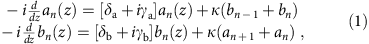where z is the evolution coordinate. The quantities an(z), bn(z) are the propagating amplitudes in both lattice sites in unit cell n, the quantities δa and δb represent the real part of the potential, and γa and γb represent the imaginary part of the potential (or gain/loss parameter), respectively. The transverse dynamics in the lattice is described by the hopping parameter (or coupling constant) κ. In the particular case of δa=δb=δ and γa=−γb=γ, the system is -symmetric, as in this case the real part of the potential is symmetric and the imaginary part is antisymmetric16,17. A sketch of such a lattice is shown in Fig. 1a. In our analysis, however, we will not restrict ourselves to the -symmetric case, but will consider a general non-Hermitian lattice with arbitrary δa,b and γa,b instead. In this case, the evolution dynamics is dictated by the spectrum of propagation constants β: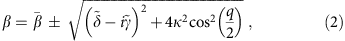where q is the transverse Bloch momentum,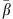=(δa+δb)/2+i(γa+γb)/2,=(δaδb)/2, and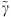=(γbγa)/2. It is noteworthy that such a class of dissipative non-Hermitian systems is said to possess broken -symmetry, as the eigenvalue spectrum is complex. Unbroken -symmetry, that is, a purely real eigenvalue spectrum, can be achieved only in lattices with δa=δb and an inhomogeneous inter-site coupling25,26.

In Fig. 1b, an example of the spectrum (equation (2)) is shown. For this arrangement, the (normalized) lattice parameters are chosen to be δa=γa=0 cm−1, δb=γb=1 cm−1 and κ=1.5 cm−1. In this figure, the solid lines indicate the upper band (the ‘+’ sign in equation (2)) and the dashed lines indicate the lower band (the ‘−’ sign). Moreover, the blue lines represent the real part of the spectrum and the red lines the imaginary part. Clearly, the imaginary part of the eigenmode’s propagation constant is a function of the transverse momentum q. Hence, eigenmodes in different regions of the spectrum will experience different losses, depending on the value of the imaginary part of their propagation constant. As a consequence, in both bands modes in the centre of the spectrum (where the lattice momentum q≈0) experience an intermediate loss, which is close to the average loss in the system. At the edge of the spectrum (around qπ) the situation is very different. There, the modes in the upper band suffer from a loss that is much higher than the systems’s average loss, whereas in the lower band the modes experience much less loss. Hence, because of decay, the modes in the upper band at the edge of the spectrum will disappear after a relatively short propagation distance z, whereas the modes in the centre of the spectrum will disappear somewhat later. Only the modes in the lower band around qπ will prevail at long propagation distances. Therefore, the spectrum will considerably be getting narrower during propagation of the wave packet along z, and only a part of the spectrum will contribute to transport.

To explore the impact of this phenomenon, we perform a numerical analysis. To this end, we solve the tight-binding equation (1) for an array of N=200 waveguides using a standard Runge–Kutta scheme and calculate the variance σ2(z) at every computation step. A simulation of the wave evolution in a -symmetric dissipative lattice is shown in Fig. 2a, where δa=δb=γa=0 cm−1, γb=2.0 cm−1 and κ=0.5 cm−1. Initially, the wave exhibits ballistic spreading as all eigenmodes still contribute to the transverse transport. However, after a certain propagation distance z the spreading clearly slows down, and the strongly modulated pattern, which is typical of ballistic transport in a periodic lattice, washes out completely. In Fig. 2b, a double-logarithmic plot shows the variance σ2(z) as a function of the gain/loss parameter γb. It is noteworthy that in the ballistic case, one finds σ2(z)~z2; plotting this with double-logarithmic axes results in a straight line with slope 2. In contrast, diffusive transport is characterized by σ2(z)~z2, which yields a straight line with slope 1 in a such a plot. Whereas one finds for γa=γb=0 cm−1 the expected ballistic spreading, for γb>0 cm−1 one clearly sees a sudden transition in the transport properties from initially ballistic (slope 2) to diffusive (slope 1). The distance, where this transition happens decreases as the loss parameter γb increases (note that γa=0 cm−1 for all graphs). We attribute this phenomenon to the narrowing of the spectrum: after some specific propagation distance zcrit, essentially only a part of the spectrum contributes to transport. In fact, the continuous spectral contraction leads to the characteristic diffusive spread of the wave packet observed in our simulations.

To understand this process beyond the intuitive spectrum-based explanation of this effect, we developed a full analytical theory of this effect, which is provided in the Methods section. Within this theory, the critical distance around which transport slows down from ballistic to diffusive is given by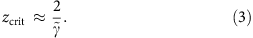Around zcrit, the spectral narrowing reaches a strength that impedes ballistic transport because of an insufficient number of propagating modes. Therefore, in essence for zzcrit the transport is ballistic, whereas for zzcrit it enters the diffusive regime. Importantly, the critical distance zcrit depends strongly on the difference in the loss parameters, and the dependence on the coupling coefficients κ is negligible. Interestingly, our simulations also indicate that zcrit slightly shifts to larger values for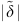>0 cm−1.

### Experiment

For the experimental demonstration of our theoretical results, we fabricated various optical waveguide samples employing the direct-laser writing technology27. A sketch of such a waveguide array is shown in Fig. 3a, and a microscopic image from the end facet of one specific realization is shown in Fig. 3b. For further details of the fabrication process, we refer the reader to the Methods section. Each sample was 10 cm long and contains 60 waveguides with an inter-site spacing of d=17 μm. These parameters were chosen such that the light does not reach the boundaries of the lattice during propagation.

To introduce additional, well-defined losses in every second guide, these sites are sinusoidally modulated transverse to the lattice plane with an amplitude of A=3 μm. The induced curvature then facilitates radiation and, therefore, enhanced losses. These losses can be tuned precisely by changing the curvature of the waveguide modulation, which we achieve by adjusting the period of the modulation at constant amplitude. In our study, depending on the specific sample, the number of periods per sample length was ranging between 23 and 103. Note that for such small modulation amplitudes of only A=3 μm, the average spacing between the waveguides is always d≈17 μm, resulting in a homogeneous coupling constant κ. However, the modulation causes a slight rise of the propagation constant in the modulated guides because of the increased optical path. This can be compensated by artificially increasing the refractive index of the unmodulated waveguides. However, the mobility transition in non-Hermitian lattices takes place also for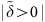; hence, in our samples we did not compensate for the additional detuning of the modulated guides.

Our experimental results are summarized in Fig. 4. To excite the entire spectrum, we launch light into the central waveguide of the lattice. An image of the propagating light beam is shown in Fig. 4a, obtained using a fluorescence microscopy technique28 (see Methods section). For small propagation distances, the typical ballistic diffraction pattern is observed, whereas for larger propagation distances the modulation of the pattern washes out and the spreading slows down. In Fig. 4b, we show a simulation of the experimental pattern with parameters δa=δb=γa=0 cm−1, γb=2 cm−1 and κ=1.1 cm−1. From the experimental fluorescence microscopy pattern in Fig. 4a, we extract the variance, which we plot using a double-logarithmic plot in Fig. 4c. The transition from ballistic to diffusive transport is clearly visible at zcrit≈2 cm: the initial ballistic spread (slope 2) suddenly slows down to diffusive (slope 1). We would like to emphasize the remarkable agreement between both the experimental and theoretical curve, as for the simulation no fitting parameters were used. The coupling parameter κ=1.1 cm−1 used in the simulation is an experimental value obtained from measurements in a single directional coupler with a waveguides separation of 17 μm. The loss parameter γb=2 cm−1 was extracted from loss measurements in isolated sinusoidally bent waveguides.

We repeat our experiments for different modulation periods in the waveguides, which results in different loss parameters γb. The position of the transition point zcrit is extracted from the fluorescence images and is plotted in Fig. 4d. As predicted by our theory, the value of zcrit is in very good agreement with equation (3). The fluorescence images and variance plots of all data points in Fig. 4a are shown in Fig. 5.

## Discussion

For the first time, we have observed the coexistence of ballistic and diffusive transport in a static, ordered system, based on non-Hermiticity. The inhomogeneous losses associated with the eigenmodes result in a contraction of the spectrum, which hinders ballistic transport because of an insufficient number of propagating modes. The transition from the initially ballistic motion to a diffusive transport is generally impossible in static Hermitian systems, where sub-ballistic transport is only possible through the introduction of disorder. Moreover, it was shown that the critical distance—where the transition of the transport regimes takes place—depends only on the degree of dissipation and not on the coupling between the waveguides.

Our theoretical and experimental work suggests various intriguing questions. In terms of applications, can a non-Hermitian waveguide lattice be used as a sophisticated mode filtering device, for any desired Bloch waves and not only those at the edge of the Brillouin zone (around q=π)? Can one design a device with real-time loss control to actively tune the width of the beam at the sample’s output facet? These issues might be of particular interest in the fields of telecommunication or quantum computing, as especially in the field of quantum optics there is a great desire to prepare single as well as entangled photons in well-defined states. Moreover, also from the more fundamental side, many interesting questions arise: what is the impact of losses in two-dimensional systems, in particular photonic graphene29? How the light behaves at defects and interfaces in lossy systems? Does disorder or nonlinearitiy facilitate or hinder transport30? Moreover, can the band structure be modified via higher-order coupling effects31 to achieve different transport regimes such as sub-diffusive, super-diffusive or even super-ballistic transport32? Our work will enable these questions, as well as many others, to be addressed.

## Methods

### Theoretical foundation

To derive the corresponding results, we start from the following system of equations: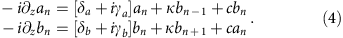The first step in analysing this system is extracting its eigenvalue spectrum. This is done by starting with the usual ansatz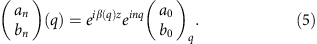Substituting this into equation (4), one arrives at an expression that determines the eigenvalues β(q) as well as the eigenvectors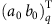: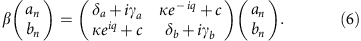Therefore, the eigenvalue spectrum is given by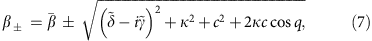where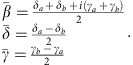The corresponding eigenvectors are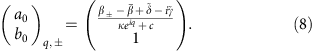As described in the paper, we are interested in the evolution of a single waveguide excitation. For this specific case, the initial conditions (at z=0) are an=δn0 and bn=0. From these initial conditions, it is straight forward to write down the evolution of the wave packet in the form of an integral: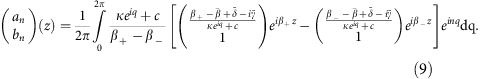Equation (9) is the full formal solution of equation (4) with the given initial conditions. However, given this form of solution it is difficult to predict a transition from ballistic to diffusive transport. On this account, the goal of this section is to derive the existence of such a transition.

As discussed in the main text, the transport regime is solely determined by the variance of the wave packet. Consequently, we calculate the variance of the wave packet given by equation (9) for large propagation distances z. For simplicity, in the following we will only consider the variance on the an sublattice. However, the derivation for the bn sublattice follows analogously to the way presented.

For simplicity, let us assume that the bn waveguides exhibit more loss than the an waveguides, that is, γba. From this it follows thatis a positive quantity. Keeping this in mind, let us take yet another look at the eigenvalue spectrum (equation (7)). It was already stated in the main text that the specific case of κ=c always leads to a complex spectrum. From here on, let us proceed with this specific case. For such a complex spectrum, the modes that exhibit the least amount of loss are those in the lower band (βq) around qπ. Hence, for large propagation distances z, one can approximate the evolution of the an by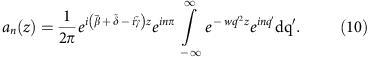To arrive at this point, first we have neglected the contribution of the modes of the upper band. Second, we have used the Taylor expansion of β± around q=π, which reads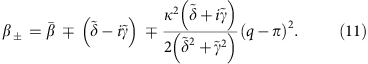Because of the dominance of the Gaussian term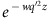, for large z one can approximate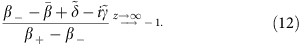In equation (10), also q′=q+π and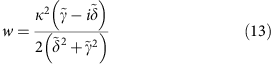have been used. The extension of the integration limits in equation (10) can be justified because of the fact that for large z, the width of the Gaussian is much smaller than the initial integration range. At this point, it needs to be emphasized that the approximation performed in equation (7) is only valid for a non-vanishing real part of w. Moreover, this condition is equivalent to the demand of a non-vanishing loss detuning.

Finally, the evaluation of the integral in equation (10) yields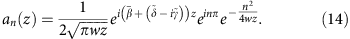Now, the last step in the analysis will be to calculate the variance of the wave packet. The variance for such a discrete and dissipative system is defined by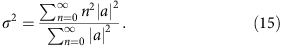Using the explicit form of an, one obtains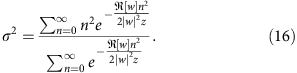For large z, the sums can be approximated by integrals using x=n/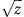and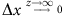. Hence, one finds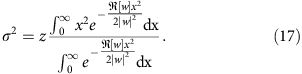Evaluating the Gaussian integral yet again, one finds the variance to be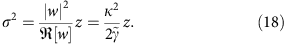This shows mathematically that the variance rises with the first power of z. Hence, the propagation of the wave packet is diffusive.

Subsequently, we want to derive the approximate region of the transition, which corresponds to the critical distance zcrit given by equation (3). For simplicity, we only regard the case of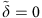. For the approximation (equation (10)) to hold, all modes outside an ε-region around q=π need to be damped sufficiently, and hence need to be smaller than some value δ. For our analysis, it is sufficient to fix δ at δ=e−2. This damping condition reads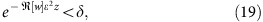or equivalently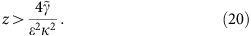It is important to note that ε cannot be chosen arbitrarily, but one has the additional condition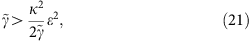which follows from the evaluation of the denominator in equation (12) and the Taylor expansion of the eigenvalues. Connecting both conditions, one obtains equation (3).

### Waveguide fabrication

Our samples are fabricated in bulk-fused silica wafers using the femtosecond laser direct-write aproach27 employing a Ti:Sapphire Mira/RegA laser system (Coherent Inc.) operating at a wavelength of 800 nm, a repetition rate of 100 kHz and a pulse length of 170 fs. The light is focused inside the sample by a × 20 microscope objective (NA=0.35), and by continuously moving the sample using a high-precision positioning system (Aerotech Inc.) the waveguides are created by the induced refractive index increase. For the fabrication of our samples, the pulse energy was adjusted to 320 nJ and the writing velocity was set to 1.5 mm s−1.

To introduce enhanced and controllable losses to specific waveguides, an additional sinusoidal modulation was applied to the waveguides to achieve additional radiation losses. The modulation is introduced into the vertical direction, as this prevents the radiated light from travelling through the lattice and being recollected by other waveguides.

### Fluorescence microscopy

For the direct monitoring of the light propagation in our samples (see Fig. 4a), we used a fluorescence microscopy technique28. A massive formation of non-bridging oxygen hole colour centres occurs during the writing process, when fused silica with a high content of hydroxide is used, resulting in a homogeneous distribution of these colour centers along the waveguides. When light from a Helium-Neon laser at λ=633 nm is launched into the waveguides, the non-bridging oxygen hole colour centres are excited and the resulting fluorescence (λ=650 nm) can be directly observed using a charge-coupled device camera with an appropriate narrow linewidth filter. As the colour centres are formed exclusively inside the waveguides, this technique yields a high signal-to-noise ratio.

How to cite this article: Eichelkraut, T. et al. Mobility transition from ballistic to diffusive transport in non-Hermitian lattices. Nat. Commun. 4:2533 doi: 10.1038/ncomms3533 (2013).

## References

1. 1

Drude, P. Zur elektronentheorie der metalle. Ann. Phys. 306, 566–613 (1900).

2. 2

Kittel, C. & Kroemer, H. Thermal Physics WH Freeman (1980).

3. 3

Ottino, J. M. & Wiggins, S. Introduction: mixing in microfluidics. Philos. Trans. Roy. Soc. Lond. Ser. A: Math. Phys. Eng. Sci. 362, 923–935 (2004).

4. 4

Stejskal, E. O. & Tanner, J. E. Spin diffusion measurements: spin echoes in the presence of a time-dependent field gradient. J. Chem. Phys. 42, 288–292 (1965).

5. 5

Schwartz, T., Bartal, G., Fishman, S. & Segev, M. Transport and anderson localization in disordered two-dimensional photonic lattices. Nature 446, 52–55 (2007).

6. 6

Rittner, A. S. C. & Reppy, J. D. Disorder and the supersolid state of solid 4He. Phys. Rev. Lett. 98, 175302 (2007).

7. 7

Holt, R. G., Clarke, C. C. & McDaniel, J. G. An acoustic levitation technique for measuring the rheology of foam near the order-disorder transition. J. Acoust. Soc. Am. 105, 1146–1146 (1999).

8. 8

Billy, J. et al. Direct observation of anderson localization of matter waves in a controlled disorder. Nature 453, 891–894 (2008).

9. 9

Roati, G. et al. Anderson localization of a non-interacting bose-einstein condensate. Nature 453, 895–898 (2008).

10. 10

Anderson, P. W. Absence of diffusion in certain random lattices. Phys. Rev. 109, 1492–1505 (1958).

11. 11

Lahini, Y. et al. Anderson localization and nonlinearity in one-dimensional disordered photonic lattices. Phys. Rev. Lett. 100, 013906 (2008).

12. 12

Amir, A., Lahini, Y. & Perets, H. B. Classical diffusion of a quantum particle in a noisy environment. Phys. Rev. E 79, 050105 (2009).

13. 13

Barthelemy, P., Bertolotti, J. & Wiersma, D. S. A lévy flight for light. Nature 453, 495–498 (2008).

14. 14

Marksteiner, S., Ellinger, K. & Zoller, P. Anomalous diffusion and lévy walks in optical lattices. Phys. Rev. A 53, 3409–3430 (1996).

15. 15

Glansdorff, P. & Prigogine, I. Thermodynamic Theory of Structure, Stability and Fluctuations (John Wiley & Sons Ltd (1971).

16. 16

Bender, C. M. & Boettcher, S. Real spectra in non-hermitian hamiltonians having PT symmetry. Phys. Rev. Lett. 80, 5243–5246 (1998).

17. 17

El-Ganainy, R., Makris, K. G., Christodoulides, D. N. & Musslimani, Z. H. Theory of coupled optical -symmetric structures. Opt. Lett. 32, 2632–2634 (2007).

18. 18

Makris, K. G., El-Ganainy, R., Christodoulides, D. N. & Musslimani, Z. H. Beam dynamics in symmetric optical lattices. Phys. Rev. Lett. 100, 103904 (2008).

19. 19

Musslimani, Z. H., Makris, K. G., El-Ganainy, R. & Christodoulides, D. N. Optical solitons in periodic potentials. Phys. Rev. Lett. 100, 030402 (2008).

20. 20

Longhi, S. Bloch oscillations in complex crystals with symmetry. Phys. Rev. Lett. 103, 123601 (2009).

21. 21

Guo, A. et al. Observation of -symmetry breaking in complex optical potentials. Phys. Rev. Lett. 103, 093902 (2009).

22. 22

Rüter, C. E. et al. Observation of parity-time symmetry in optics. Nat. Phys. 6, 192–195 (2010).

23. 23

Regensburger, A. et al. Parity-time synthetic photonic lattices. Nature 488, 167–171 (2012).

24. 24

Yariv, A. Optical Electronics 4th edn (Saunders College Publishing, 1991).

25. 25

Zheng, M. C., Christodoulides, D. N., Fleischmann, R. & Kottos, T. optical lattices and universality in beam dynamics. Phys. Rev. A 82, 010103 (2010).

26. 26

Dmitriev, S. V., Sukhorukov, A. A. & Kivshar, Y. S. Binary parity-time-symmetric nonlinear lattices with balanced gain and loss. Opt. Lett. 35, 2976–2978 (2010).

27. 27

Szameit, A. & Nolte, S. Discrete optics in femtosecond-laser-written photonic structures. J. Phys. B 43, 163001 (2010).

28. 28

Szameit, A. et al. Quasi-incoherent propagation in waveguide arrays. Appl. Phys. Lett. 90, 241113 (2007).

29. 29

Szameit, A., Rechtsman, M. C., Bahat-Treidel, O. & Segev, M. -symmetry in honeycomb photonic lattices. Phys. Rev. A 84, 021806 (2011).

30. 30

Jovic, D. M., Denz, C. & Belic, M. R. Anderson localization of light in -symmetric optical lattices. Opt. Lett. 37, 4455–4457 (2012).

31. 31

Szameit, A. et al. Long-range interaction in waveguide lattices. Phys. Rev. A 77, 043804 (2008).

32. 32

Levi, L., Krivolapov, Y., Fishman, S. & Segev, M. Hyper-transport of light and stochastic acceleration by evolving disorder. Nat. Phys. 8, 912–917 (2012).

## Acknowledgements

We acknowledge support by the German Ministry of Education and Research (Center for Innovation Competence program, Grant 03Z1HN31) and the Thuringian Ministry for Education, Science and Culture (Research group Spacetime, Grant no. 11027-514).

## Author information

Authors

### Contributions

T.E. and A.S. developed the theory. T.E. and R.H. performed the simulations. T.E. and F.D. carried out the experimental work. A.S. supervised the project. All authors co-wrote the paper.

### Corresponding author

Correspondence to A. Szameit.

## Ethics declarations

### Competing interests

The authors declare no competing financial interests.

## Rights and permissions

Reprints and Permissions

Eichelkraut, T., Heilmann, R., Weimann, S. et al. Mobility transition from ballistic to diffusive transport in non-Hermitian lattices. Nat Commun 4, 2533 (2013). https://doi.org/10.1038/ncomms3533

• Accepted:

• Published:

• ### Coexistence of dynamical delocalization and spectral localization through stochastic dissipation

• Sebastian Weidemann
• , Mark Kremer
• , Stefano Longhi
•  & Alexander Szameit

Nature Photonics (2021)

• ### Controllable unidirectional transport and light trapping using a one-dimensional lattice with non-Hermitian coupling

• Lei Du
• , Yan Zhang
•  & Jin-Hui Wu

Scientific Reports (2020)

• ### Effect of loss on transverse localization of light in 1D optical waveguide array in the presence of Kerr-type nonlinearity

• , D. Mirshamsi
•  & H. Rastegar Moghaddam Rezaeiun

Optical and Quantum Electronics (2020)

• ### Demonstration of a two-dimensional $${\cal P}{\cal T}$$ P T -symmetric crystal

• Mark Kremer
• , Tobias Biesenthal
• , Lukas J. Maczewsky
• , Matthias Heinrich
• , Ronny Thomale
•  & Alexander Szameit

Nature Communications (2019)

• ### Observation of PT-symmetric quantum interference

• F. Klauck
• , L. Teuber
• , M. Ornigotti
• , M. Heinrich
• , S. Scheel
•  & A. Szameit

Nature Photonics (2019)